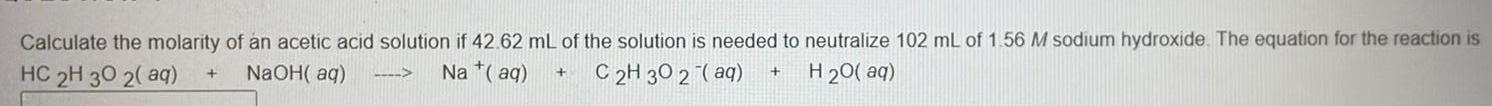Question:

# Calculate the molarity of an acetic acid solution if 42.62

Last updated: 8/4/2022Calculate the molarity of an acetic acid solution if 42.62 mL of the solution is needed to neutralize 102 mL of 1.56 M sodium hydroxide. The equation for the reaction is HC2H3O2(aq) +NaOH(aq)⇒Na+(aq)+C2H302-(aq)+H20(aq)